# the values of h ( − 2 ) , h ( 0 ) , h ( 2 ) and h ( 3 )### Precalculus: Mathematics for Calcu...

6th Edition
Stewart + 5 others
Publisher: Cengage Learning
ISBN: 9780840068071### Precalculus: Mathematics for Calcu...

6th Edition
Stewart + 5 others
Publisher: Cengage Learning
ISBN: 9780840068071

#### Solutions

Chapter 2.3, Problem 5E

a.

To determine

## To calculate: the values of h(−2),h(0),h(2) and h(3)

Expert Solution

h(2)=1h(0)=1h(2)=3h(3)=4

### Explanation of Solution

Given information: the graph of function h is provided,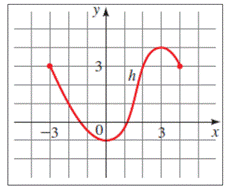Graph: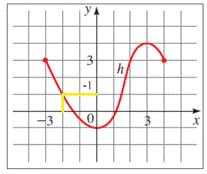Interpretation:

In the graph, the value of h(2) is the corresponding y- value at x = 2 , that is,

h(2)=1

Similarly, the corresponding y -values for,

h(0) at x = 0 is 1

h(2) at x = 2 is 3

h(3) at x = 3 is 4

Hence,

h(2)=1h(0)=1h(2)=3h(3)=4

b.

To determine

### To calculate: the domain and the range of the provided graph

Expert Solution

The domain and range of h is [-3,4] and [-1,4], respectively.

### Explanation of Solution

Given information: the graph of function h is provided,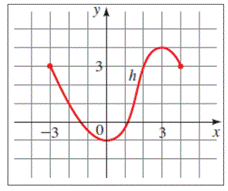Graph: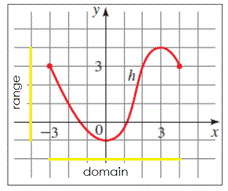Interpretation:

The domain of the function h is all the ix-values of the points on the graph, and the range is all the corresponding y -values.

From the graph of h , we see that the domain of h is the interval [-3, 4] and the range of h is the interval [-1, 4].

Hence, the domain and range of h is [-3,4] and [-1,4], respectively.

c.

To determine

### To calculate: the value of x for which h ( x ) = 3

Expert Solution

the value of x for which h ( x ) = 3 is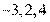### Explanation of Solution

Given information: the graph of function h is provided,Graph: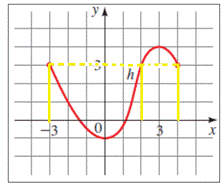Calculation:

The function value of h ( a ) from the graph of h , is the height of the graph above the x -axis at x = a ,

Therefore, h ( x ) = 3 is the x -value on the x -axis where the height above it is 3, that are,

h(3)=3h(2)=3h(4)=3

Hence, the value of x for which h ( x ) = 3 is 3,2,4 .

d.

To determine

### To calculate: the values for h(x)≤3

Expert Solution

the values of x for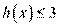areand x = 4

### Explanation of Solution

Given information: the graph of function h is provided,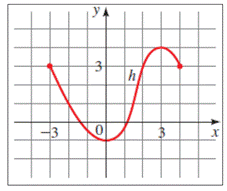Calculation:

When h(x)3 is expressed in words it means, h ( x ) is less than equal to 3. From part c.,

h(3)=3h(2)=3h(4)=3

Therefore, the x-values from the graph for h(x)3 ,

h(3),h(2),h(1),h(0),h(2),h(4)

Hence, the values of x for h(x)3 are 3x2 and x = 4

### Have a homework question?

Subscribe to bartleby learn! Ask subject matter experts 30 homework questions each month. Plus, you’ll have access to millions of step-by-step textbook answers!